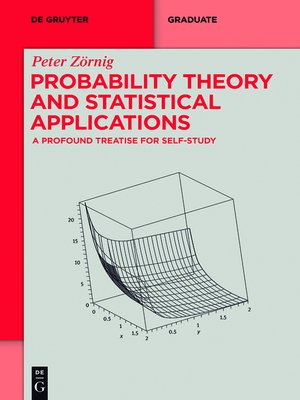# Probability Theory and Statistical Applications

## ebook ∣ A Profound Treatise for Self-Study · De Gruyter Textbook

### By Peter ZörnigWith an OverDrive account, you can save your favorite libraries for at-a-glance information about availability. Find out more about OverDrive accounts.

### Title found at these libraries:

This accessible and easy-to-read book provides many examples to illustrate diverse topics in probability and statistics, from initial concepts up to advanced calculations. Special attention is devoted e.g. to independency of events, inequalities in probability and functions of random variables. The book is directed to students of mathematics, statistics, engineering, and other quantitative sciences, in particular to readers who need or want to learn by self-study. The author is convinced that sophisticated examples are more useful for the student than a lengthy formalism treating the greatest possible generality.

Contents:
Mathematics revision
Introduction to probability
Finite sample spaces
Conditional probability and independence
One-dimensional random variables
Functions of random variables
Bi-dimensional random variables
Characteristics of random variables
Discrete probability models
Continuous probability models
Generating functions in probability
Sums of many random variables
Samples and sampling distributions
Estimation of parameters
Hypothesis tests